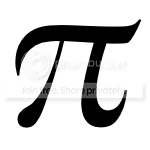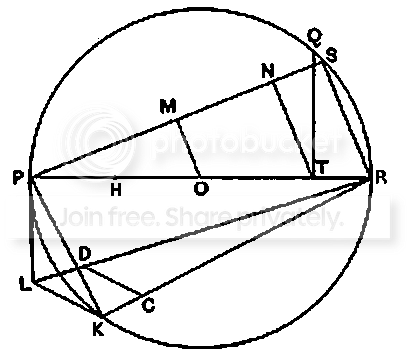0

## Messing With Pi

Published on Thursday, February 07, 2008 in , , , ,Did you ever receive that e-mail that claims that some state's legislature (usually Alabama) has recently passed a law redefining Pi? As Snopes notes, this is false. However, 111 years ago this week, the Indiana state legislature came very close to doing just that.

The change wasn't done to make Pi consistent with the Bible, but rather to deal with the age-old problem of trying to construct a square with the same area as a given circle, otherwise known as squaring the circle. Because Pi is transcendental, we now know that this is impossible.

However, what if you were working before the 19th century, before this was proved, and before calculus? There are numerous ways to get very, very close to the circle, and it's not unreasonable to think that just a few more adjustments would be needed to perfect the process. Let me show you how the madness takes hold. One of the best approaches I've found for seemingly squaring the circle was detailed by Stu Savory in April, 2005 (26 days too late if you ask me). He does a good job explaining, but I'd like to break it down a little differently for my readers. It uses only a compass and a straightedge.We start by working on the line that will become the circle's diameter. Draw a horizontal line, and mark the rightmost end as R. Open the compass to a width of an inch or so, place the spike of the compass at R, and mark point T on the line to the left of R. Now place the spike on T, and mark a point to the left of T (not labeled). Place the spike on this unlabeled point, and mark one more point to its left, which will be labeled O. In short, OT should be exactly twice as long as RT.

Now, open your compass to length OR, with the spike at O. Use the compass to draw a circle. Mark as P the point where the horizontal line intersects with the circle on the left. Our final step concerning the diameter line itself (POR) is to bisect OP (Java required), marking OP's midpoint as H.

Next, we're going to make some measurements above POR. Construct a vertical line from point T. Mark the point at which this line intersects the circle as Q. Using the same length as QT, draw a chord starting at R. Mark the other end of this chord as S (length of QT=length of RS). Draw a line from S to P. Construct a line parallel to RS through point T (Java required), marking the point where it intersects SP as N. then construct another line parallel to RS through point O, marking the point where it intersects SP as M.

Finally, we're going to work below PQR, and get our square's base measurement. Set your compass open to length PM, with the spike at P. Swing the compass to mark the point K, below POR, on the circle's circumference, and draw the PK chord (PK=PM). Set your compass to the length of MN, and then construct a tangent at a right angle to POR, marking the bottom-most point of this tangent as L. Draw lines RL, RK and KL. Finally, construct a line parallel to LK, going through point C. Mark as D the point where this line intersects RL.

So what does this give us? RD is the base of our square we need. Let's work through this next part slowly.

The formula for the area of the circle, as we all learned in school, is Pi * r2. The formula for a square with a base of x is x2. Because of the way in which these measurements were done, RD will always be a straight line about 1.772453 times longer than RO, the circle's radius. As it happens, this is the square root of Pi!

In other words, the length of line RD equals the circle's radius times the square root of Pi ((sqrt(pi))*r). Using RD as the base of a square, we can get the square's area by multiplying this by itself! Squaring the square root of Pi gives us Pi, of course, and squaring r gives us r2, or Pi * r2 for the area of this square!

Everything seems right, but can you figure out why this isn't a true squaring of the circle? What actually winds up happening is that RD is the square root of 355/113, a common rational fraction used for Pi early on. 355/113=3.1415929204, which is accurate to 6 places after the decimal point! It's square root is 1.7724539262, which also accurate to 6 places after the decimal point. This gives a square and a circle that are so close in area that, using a pencil that could mark in 1/100 of an inch, you would have to create a circle more than 4 miles in diameter to see your error!

If you look at the bottom of Stu Savory's blog entry, he has a quicker and more interesting way of apparently squaring the circle with a compass, straightedge and a coin!

Are you beginning to see why so many people were obsessed with squaring the circle? When you don't know that Pi goes on forever, it seems to be a matter of just making a few more adjustments to make a historical mathematical breakthrough! This was actually so common that, at one point, the insane belief that one could square a circle was listed in medical texts as morbidus cyclometricus!

I hope you've enjoyed this rather odd look at a weird bit of mathematical history.